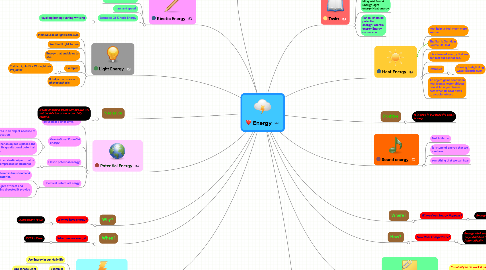# Energy

Get Started. It's FreeEnergy## 1. How?

### 1.1. How Does Energy Work?

1.1.1. Energy Work In Many Different Ways And Also It Happens Automatically.

## 3. Heat Energy

### 3.4. Examples

3.4.1. Burning fuels,Boiling water,Electric oven

## 6. Light Energy

### 6.4. Examples

6.4.1. Traffic Lights,Fire Works,Movie Projector

## 7. Kinetic Energy

### 7.3. Examples Of Kinetic Energy

7.3.1. kayaking,ironing,running,writting

## 9. Potential Energy

### 9.2. Gravitational Potential Energy

9.2.1. Stored in an object because of its location.

9.2.1.1. Parachuting,Skydiving

9.2.2. The higher an object is placed,the greater its gravitational potential energy.

### 9.3. Elastic potential energy

9.3.1. Stored in an elastic object that is being compressed or stretched.

9.3.1.1. Archery,Jack in the box

### 9.4. Chemical Potential Energy

9.4.1. Stored in fuels,food and batteries.

9.4.2. Fuels can be burnt to give off heat and light.When food is being digested,It provide us with energy.

## 10. Why?

### 10.1. Why we have energy?

10.1.1. is Important To Us.

## 11. Where?

### 11.1. Where Does Energy Happens?

11.1.1. Energy Happens EVERY Where.

## 12. When?

### 12.1. When we use energy?

12.1.1. EVERY Time.

## 15. Electrical Energy

### 15.2. Examples

15.2.1. Appliances,Light

## 16. Energy Conversions

### 16.2. Examples

16.2.1. When you throw a basketball,it moves and gains kinetic energy.A rice plant converts light energy from the Sun into chemical potential energy during photosynthesis.